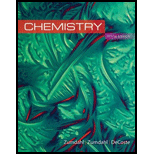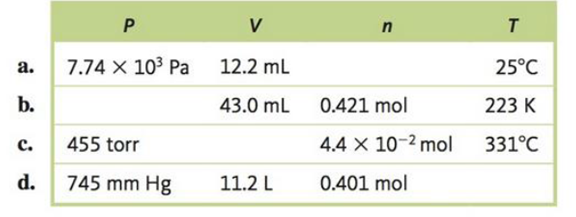# Complete the following table for an ideal gas.### Chemistry

10th Edition
Steven S. Zumdahl + 2 others
Publisher: Cengage Learning
ISBN: 9781305957404

#### Solutions

Chapter
Section### Chemistry

10th Edition
Steven S. Zumdahl + 2 others
Publisher: Cengage Learning
ISBN: 9781305957404
Chapter 5, Problem 52E
Textbook Problem
534 views

## Complete the following table for an ideal gas.Interpretation Introduction

Interpretation:

From the given table, missed values of the temperature, pressure, volume and number of moles of an ideal gas should be determined on the basis of ideal gas equation.

Concept introduction:

By combining the three gaseous laws namely Boyle’s law, Charles’s law and Avogadro’s law a combined gaseous equation is obtained. This combined gaseous equation is called Ideal gas law.

According to ideal gas law,

PV=nRT

Where,

P = pressure in atmospheres

V= volumes in liters

n = number of moles

R =universal gas constant ( 0.08206L×atm/K×mol )

T = temperature in kelvins

By knowing any three of these properties, the state of a gas can be simply identified with applying the ideal gas equation.

### Explanation of Solution

(a)

To determine: the number of moles of an ideal gas in the row a from the rest of the other values.

According to ideal gas equation,

PV=nRT

From the given data to calculate the unknown temperature,

P = 7.74×103Pa , Since

1 atm=1.013 ×105Pap=7.74×103Pa×1atm1.013 ×105Pa=0.0764atm

V =12.2 Ml,   since

1L=1000mL

V=12.2mL×1L1000mL=0.0122L

n =?

R =universal gas constant ( 0.08206L×atm/K×mol )

T = 25°C or 25+273=298 K       since, 273+°C=K

From the ideal gas equation, number of moles can be calculated as follows;

That is,        PV=nRT

Then,             n=PVRT

By adding the given values in the above equation the number of moles can be determined,

So, the above equation becomes,

n=0.0764atm×0.0122L0.08206L×atm/K×mol×298K=3.81×10-5mol

(b)

To determine: the pressure of an ideal gas in the row b from the rest of the other values

According to ideal gas equation,

PV=nRT

From the given data to calculate the unknown temperature,

P =?

V =43.0 mL

1L=1000mLV=43.0mL×1L1000mL=0.0430L

n = 0.421mol

R =universal gas constant ( 0.08206Latm/Kmol )

T = 223K

From the ideal gas equation, the unknown volume can be calculated as follows;

That is,        PV=nRT

Then,

P=nRTV

By adding the given values in the above equation the pressure of an ideal gas equation can be determined,

So, the above equation becomes,

P=0.421mol×0.08206L×atm/K×mol ×223K0

### Still sussing out bartleby?

Check out a sample textbook solution.

See a sample solution

#### The Solution to Your Study Problems

Bartleby provides explanations to thousands of textbook problems written by our experts, many with advanced degrees!

Get Started

Find more solutions based on key concepts
Which of these foods should form the bulk of the pregame meal? a. breads, potatoes, pasta, and fruit juices. b....

Nutrition: Concepts and Controversies - Standalone book (MindTap Course List)

When would irrigation be necessary at this site?

Fundamentals of Physical Geography

Understanding Nutrition (MindTap Course List)

What is population genetics?

Human Heredity: Principles and Issues (MindTap Course List)

How does the CNO cycle differ from the proton-proton chain? How is it similar?

Horizons: Exploring the Universe (MindTap Course List)

Describe four of the nutritional benefits of human milk to the infant.

Nutrition Through the Life Cycle (MindTap Course List)

Why are abyssal plains relatively rare in the Pacific?

Oceanography: An Invitation To Marine Science, Loose-leaf Versin

Review. Two constant forces act on an object of mass m = 5.00 kg moving in the xy plane as shown in Figure P7.4...

Physics for Scientists and Engineers, Technology Update (No access codes included)